﻿ Common Fixed Point Theorem in Cone Metric Spaces

Common Fixed Point Theorem in Cone Metric Spaces

K. PrudhviOPEN ACCESSPEER-REVIEWED

Common Fixed Point Theorem in Cone Metric Spaces

Department of Mathematics, University College of Science, Saifabad, Osmania University, Hyderabad, Telangana State, India

Abstract

In this paper, we study the existence of coincidence points and a unique common fixed point theorem for three self-mappings in cone metric spaces, where the cone is not necessarily normal. This result extends and improves recent related results in the literature.

• Prudhvi, K.. "Common Fixed Point Theorem in Cone Metric Spaces." American Journal of Applied Mathematics and Statistics 3.2 (2015): 68-70.
• Prudhvi, K. (2015). Common Fixed Point Theorem in Cone Metric Spaces. American Journal of Applied Mathematics and Statistics, 3(2), 68-70.
• Prudhvi, K.. "Common Fixed Point Theorem in Cone Metric Spaces." American Journal of Applied Mathematics and Statistics 3, no. 2 (2015): 68-70.

 Import into BibTeX Import into EndNote Import into RefMan Import into RefWorks

1. Introduction

Huang and Zhang  generalized the concept of a metric space, replacing the set of real numbers by an ordered Banach space. They proved some fixed point theorems of contractive type mappings over cone metric spaces. Subsequently, many authors generalized their fixed point theorems to different types using with and without normality (see, e.g., [1, 2, 3, 6, 7, 8]). Recently, Guangxing Song et.al.  obtained a new common fixed point theorems for two maps in cone metric spaces, and omitting the assumption of normality. In this paper, we proved a fixed point theorem for three maps in cone metric spaces. Our result extends and improves the results of .

We recall some definitions and properties of cone metric spaces due to Huang and Zhang .

Definition 1.1. Let E be a real Banach space and P a subset of E .The set P is called a cone if and only if:

(a). P is closed, non –empty and P ≠ {0};

b). a,b∈ℝ, a,b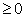, x,y∈P implies ax+by∈P;

(c). x∈P and -x∈P implies x = 0.

Definition 1.2. Let P be a cone in a Banach space E, define partial ordering ‘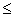’ with respect to P by xy if and only if y-x∈P. We shall write x<y to indicate x≤y but x ≠ y while x<<y will stand for y-x∈Int P, where Int P denotes the interior of the set P. This cone P is called an order cone.

Definition 1.3. Let E be a Banach space and P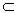E be an order cone .The order cone P is called normal if there exists L>0 such that for all x, y∈E,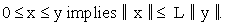The least positive number L satisfying the above inequality is called the normal constant of P.

Definition 1.4. Let X be a nonempty set of E. Suppose that the map d: X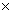X→ E satisfies:

(d1). 0 ≤ d(x, y) for all x, y∈X and

d(x,y) = 0 if and only if x = y;

(d2). d(x, y) = d(y, x) for all x, y∈X;

(d3). d(x, y)d(x, z)+d(z, y) for all x, y, z∈X.

Then d is called a cone metric on X and (X, d) is called a cone metric space.

It is clear that the concept of a cone metric space is more general than that of a metric space.

Example 1.5.  Let E =R2, P = {(x, y)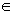E such that : x, y ≥ 0}⊂ R2, X = R and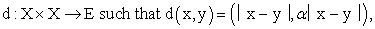where α ≥ 0 is a constant . Then (X, d) is a cone metric space.

Definition 1.6. Let (X, d) be a cone metric space .We say that {xn} is

(i) a Cauchy sequence if for every c in E with c>>0, there is N such that for all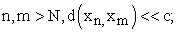(ii) convergent sequence if for any c>>0, there is an positive integer N such that for all n>N, d(xn, x) <<c, for some fixed x in X .We denote this xn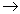x (as n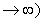.

The space (X, d) is called a complete cone metric space if every Cauchy sequence is convergent ().

Definition 1.8.  For the mapping f, g: X→X. If w = fz = gz for some z in X, then z is called a coincidence point of f and g and w is called a point of coincidence of f and g.

Definition 1.7. Let f, g: X→ X. Then the pair (f, g) is said to be (IT)-commuting at z∈X if f(g(z))=g(f(z)) with f(z)=g(z).

2. Main Result

In this section, we proved a fixed point theorem for three self- mappings in cone metric spaces and without assuming the normality.

Theorem 2.1. Let (X, d) be a cone metric space and P an order cone and f, g,h: X→X be self-maps satisfying the following condition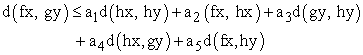(1)

for all x, y∈X, where ai≥0 (i= 1,2,3,4,5) be constants ( a1+a2+a3+2a4+a5 <1) .

If f(X)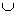g(X)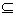h(X) and h(X) is a complete subspace of X. Then the maps f,g and h have a coincidence point p in X. Moreover if (f, h) and (g, h) are (IT)-Commuting at p, then f, g and h have a unique common fixed point.

Proof. Suppose x0 is an arbitrary point of X, and define the sequence {yn} in X

such that y2n = fx2n = hx2n+1 ,

and y2n+1 = gx2n +1= hx2n+2 , for all n = 0,1,2,3,…..

By (1), we have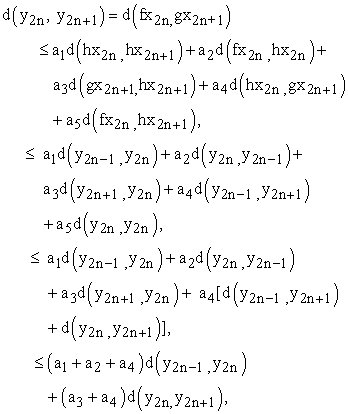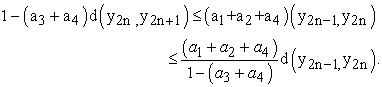Put,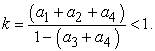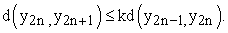(2)

Similarly it can be shown that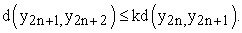Therefore, for all n,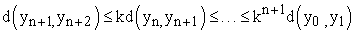Now, for any m>n,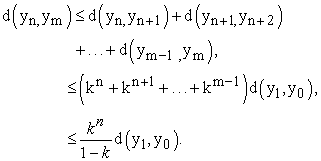Let 0<<c be given. Choose δ>0 such that c+ Nδ (0)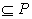, where Nδ (0)={xE:║x║<δ}.

Also choose a natural number N1 such that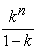d(y1 , y0)Nδ (0), for all n ≥ N1.

Thend(y1 , y0)<<c , for all n ≥ N1.

Thus, d(yn, ym)≤d(y1 , y0)<<c, for all m>n.

Therefore, {yn} is a Cauchy sequence. Since h(X) is complete, there exists q in h(X) such that h(p)=q. We shall show that hp = fp = gp. Note that d(hp,fp) = d(q,fp).

Let us estimate d(hp, fp).We have by (1) and the triangle inequality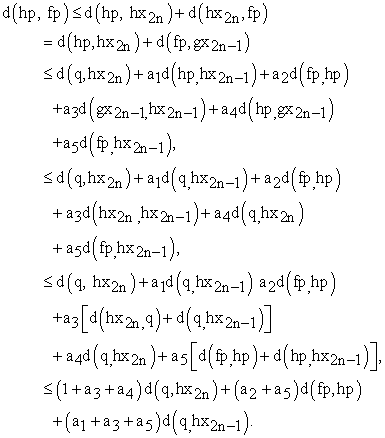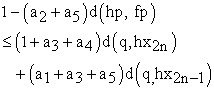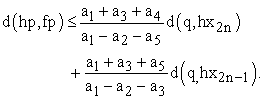(3)

Suppose 0<<c and there exists n0N such that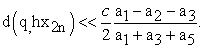(4)

and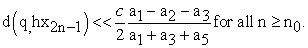(5)

From (4), (5) and (3) it follows that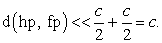And hence, d(hp, fp)<<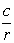for every rN.

Since,- d(hp, fp)int P, and P is closed, then as r→∞ we have that - d(hp, fp)P. Since d(hp, fp)>0, therefore d(hp, fp)P and so d(hp, fp)P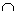(-P) ={o}.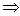d(hp, fp) = 0.

Hence, d(hp, fp) = 0.

Similarly, we can show that hp = gp.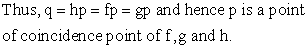(6)

Since, (f, h),(g, h) are (IT)-Commuting at p. We get by (6) and (1)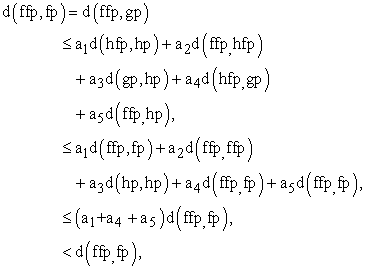since a1+ a4 + a5<1, a contradiction.

Therefore, ffp = fp. fp = ffp = fhp = hfp.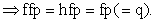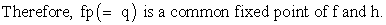(7)

Similarly , we can get that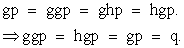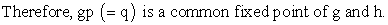(8)

Since, fp = gp = q.

Therefore from , (7) and (8) it follows that f, g, and h have a common fixed point namely q.

Uniqueness: Let q1 is another fixed point of f, g and h, then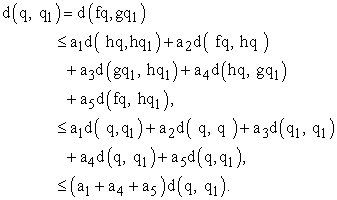Hence, d(q, q1) = 0 and so, q = q1.

Therefore, f, g, and h have a unique common fixed point.

Remark 2.2. If we choose h = g and g = f in the above Theorem 2.1, then we obtain the Theorem 2.1 of .

References

  M. Abbas and G. Jungck, Common fixed point results for nonIn article CrossRef  commuting mappings without continuity in cone metric spaces. J. Math. Anal. Appl. 341(2008) 416-420.In article CrossRef  M.Amari, D.El Moutawakil, Some new common fixed point theorems under strict contractive conditions, J. Math. Anal. Appl. 270(2002)181-188.In article  M.Arshad, A.Azam, P.vetro, Some common fixed point results on cone metric spaces, Fixed Point Theory Appl. (2009)11, Article ID 493965.In article CrossRef  Guangxing Song, Xiaoyan Sun, Yian Zhao, Guotao Wang, New common fixed point theorems for maps on cone metric spaces, Appl. Math. Lett. 32(2010)1033-1037.In article CrossRef  L.G.Huang, X.Zhang, Cone metric spaces and fixed point theorems of contractive mappings, J.Math. Anal. Appl. 332(2) (2007) 1468-1476.In article CrossRef  D. IIic, V.Rakocevic, Common fixed points for maps on cone metric space, J. Math. Anal. Appl.341(2008) 876-886.In article CrossRef  S. Rezapour and Halbarani, Some notes on the paper cone metric spaces and fixed point theorem of contractive mappings, J. Math. Anal. Appl. 345(2008), 719-724.In article  B.E.Rhoars, A comparison of various definitions of contractive mappings, Trans. Amer. Math.Soc. 26(1977)257-290.In article